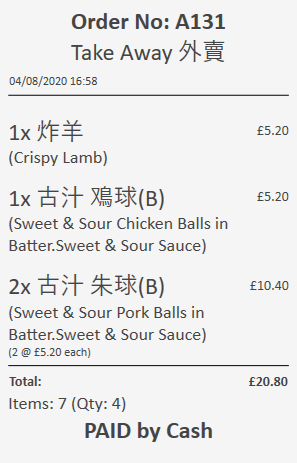# Counting product tag on ticket

I am trying to implement an item count on the kitchen ticket, so the staff know how many physical items need to go in a bag. It isn’t just the quantity, as some dishes may consist of 2 or more “items” so must be counted as such.

I have added a Custom Product Tag for `Item Count` to store the number of items each product consists of.

I can get it to show the sum of this on the ticket as follows:

``````[=F('{ORDER DETAILS:MT.Item Count.Sum}','0')]
``````

However, the above does not take the quantity into account - it just takes as each order with 1 quantity. I’ve tried

``````[=F('{ORDER DETAILS:(MT.Item Count * O.Quantity).Sum}','0')]
``````

But that just returns nothing.

I wonder if anyone else has came across needing to do this and has a quick solution?

Maybe try separating the two fields into seperate tn expressions with the *? :-/

That won’t work, because what we have is like this:

``````Item Count   Qty   Total
1            1     1
2            1     2
2            2     4
------------------------
5            4     7
``````

So we need it to show 7, but right now it will show 5 (total of individual `Item Count` values). If I just took the sum of `Item Count` and added the sum of `Quantity`, I would get `5+4=9`. So what I need is for each row, the 2 values multiplied (Item Count * Quantity) then the sum of that new value.

I am on the phone now but wouldn’t MT.Tag.count be the right syntax?

What if you kept a running total as orders are added, using program settings?

No, that will give the `Count` as the number of records and also would not consider the quantity of the order.

The data is already stored on the ticket so its just really finding the way to access it as a sum value taking quantity into account. This would be more like an overworked way of doing it and would have to handle lots of extra scenarios like when you change tickets having to recount, etc. All I need is to get the value including each item count being multiplied by quantity.

Ok I got it working now``````[=F('{ORDER DETAILS:([MT.Item Count]*[O.Quantity]).Sum}','0')]
``````

By putting the values in `[ ]` means they get processed on SQL Server rather than in SambaPOS. I knew it would be related to doing this but I couldn’t remember the syntax and took a lot of trial and error searching various things until I found an example.

Here is an example ticket, based on the table I used above. Both the Chicken Balls and Pork Balls have `Item Count=2` (they come with the sauce separate), so you can see here with the expression it correctly shows the total items as 7.5 Likes